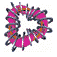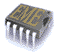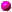## Mathematical Modeling in Nonlinear PhysicsOptical pulses are used in fiber optics technology to code bit signals in data streams. Optical pulses are transmitted through a long-haul multi-channel telecommunication networks. Multiple factors, such as polarization mode dispersion, temporal instabilities, Gordon-Haus time jitting effects, four-wave mixing and others, lead to degradation of the bit-noise ratio between optical signals and accumulative disturbances in the network. These factors become dominant obstacles for the use of optical pulses in transoceanic telecommunication lines that extend over thousand miles. New revolutionary upgrading technologies, dispersion and nonlinearity management, are designed to reduce the degradation factors.Nonlinear periodic structures or simply photonic gratings are small-sized devices used in photonic optics for all-optical ultra-fast signal processing. Optical limiters, switches and logic gates provide prospective basis for complex operations with optical signals. New principles in design of such optical gratings are based on nonlinear compensation of the refractive index. This pioneer technology guarantees stability of the photonic devices and their uniform behavior suitable for signal processing.

Mathematical modeling in nonlinear physics is focused at the three central problems:

• existence of stationary solutions in optical modules
• linear stability in the time evolution problem
• nonlinear transmission and interaction of optical signals

Research tools for solving these problems include analysis of differential equations (exact integration, asymptotic methods, numerical solutions), stability analysis (eigenvalue problems, linear algebra methods), and numerical modeling of partial differential equations (split-step, Fourier, Runge-Kutta, finite differences).Light Transmission in Photonic GratingsSoliton Propagation in Discrete Lattices